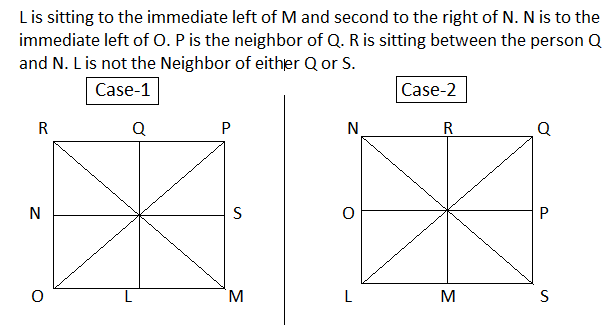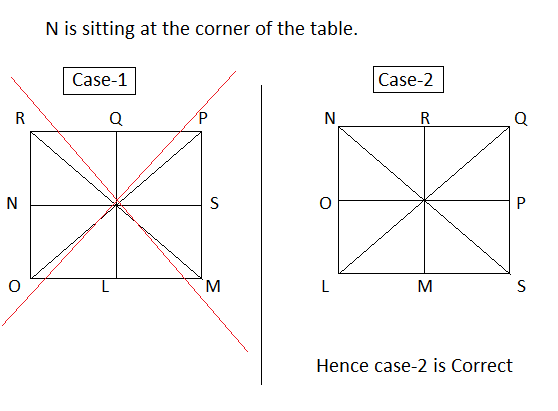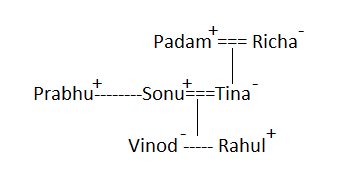# Reasoning Ability Quiz: 13 March 2021

Updated Sat, 13 Mar 2021 03:48 AM IST

Reasoning Ability Quiz: 13 March 2021

Direction: Study the following information carefully and answer the given question.

There are eight person L, M, N, O, P, Q, R, and S  Are sitting around a square table but not necessarily in the same order. Four of them are sitting at the corner and the remaining is sitting on the middle side of the table.
L is sitting to the immediate left of M and second to the right of N. N is to the immediate left of O. P is the neighbor of Q. R is sitting between the person Q and N.Source: safalta.com

L is not the Neighbor of either Q or S. N is sitting at the corner of the table.

Q-1) Who among the following sits second to the left of P?
1. L
2. S
3. M
4. R
5. N
Q-2) What is the position of N with respect to L ?
1. Third to the left
2. Second to the left
3. Third to the right
4. Immediate right
5. Immediate left
Q-3) Four of the following five are alike in a certain way and so form a group. Which is the one that does not belong to the group?
1. N
2. Q
3. S
4. L
5. R
Q-4) How many person sits between N and S, when count in clock wise direction?
1. One
2. Two
3. Three
4. Four
5. None of these
Q-5) Which of the following person sits immediate left of M?
1. L
2. S
3. P
4. O
5. None of these
Q: 6-8)
Direction: Study the following information carefully and answer the given question.
There are seven member in a family.

### Free Demo Classes

In which there were only two married couple in the family. Each couple has either One or two children. Prabhu is the brother-in-law of Tina Who is the mother of Rahul. Padam is father-in-law to Sonu. Richa is the grandmother of Vinod. Sonu and vinod were of same gender, and Rahul gender is different from Vinod.

Q-6)  How is Padam related to Rahul ?
1. Grandson
2. Granddaughter
3. Father-in-law
4. Grandfather
5. Cannot be determine
Q-7) If Prabhu is married to Priya. Then how priya is related Sonu ?
1. Brother-in-law
2. Sister-in-law
3. Son-in-law
4. Daughter-in-law
5. Mother
Q-8) How is sonu related to Padam.
1. Brother-in-law
2. Sister-in-law
3. Son-in-law
4. Daughter-in-law
5. Father
Direction: Study the following information carefully and answer the given question.
3  R  S  7  A  !  V  #  A  Q   W   1   \$   F   6   T   Z   @   X   2   A   O   U   5   *   E   L   4  E  7  %  M  A  B
Q-9 ) Which of the following element is the sixth to the left of the nineteen element from the left end of the above arrangement?
1. \$
2. 1
3. F
4. 6
5. W
Direction: Study the following information carefully and answer the given question.
3  R  S  7  A  !  V  #  A  Q   W   1   \$   F   6   T   Z   @   X   2   A   O   U   5   *   E   L   4  E  7  %  M  A  B
Q-10) which of the following element is in the middle of 8th element from the left end and 9th element from the right end ?
1. @
2. T
3. Z
4. 6
5. 2

Solution:

ANS 1-5)ANS-1) - C)M

Ans-2)  Second to the left

ANS-3)  E) R

Ans-4) – Three

ANS-5) A) L

ANS   6-8)Ans 6) - Padm is maternal grandfather of  Rahul
Ans –7)  B) sister in law
Ans-8)   Son-in-law

ANS- 9)  A) \$
Solution:
3  R  S  7  A  !  V  #  A  Q   W   1   \$   F   6   T   Z   @   X   2   A   O   U   5   *   E   L   4  E  7  %  M  A  B
19th element from the left end  =   X
6th element from the left of X = \$
Hence the correct answer is \$

ANS- 10) C) Z
Solution:
8th element from the left end = #
9th element from the right end = E
The remaining sequence is –
A  Q   W   1   \$   F   6   T   Z   @   X   2   A   O   U   5   *
Hence the middle element of this sequence is  = Z
Hence the correct answer is – Z